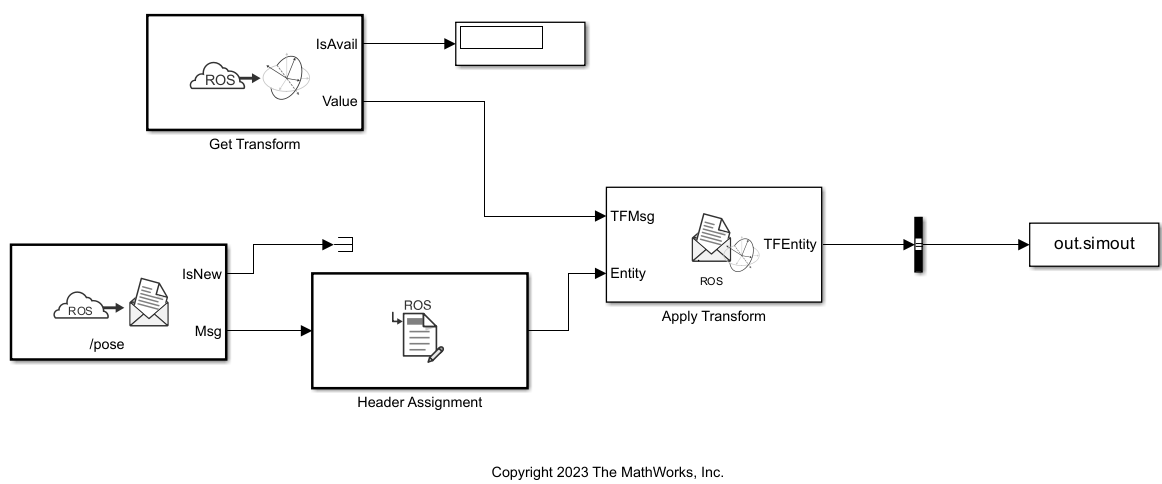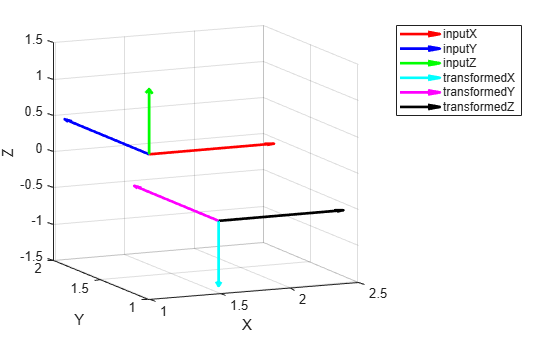This example shows how to read transformations from ROS network and use them to transform a pose message using Simulink®.

### Publish Transformation and Pose Messages on ROS Network

Initialize the ROS network.

`rosinit`
```Launching ROS Core... Creating Python virtual environment for ros1.Done. Adding required Python packages to virtual environment.Done. Copying include folders.Done. Copying libraries.Done. ......Done in 7.2019 seconds. Initializing ROS master on http://172.21.16.85:60776. Initializing global node /matlab_global_node_64587 with NodeURI http://ah-avijayar:54441/ and MasterURI http://localhost:60776. ```

Use the `exampleHelperROSStartTfPublisher` function to start publishing transformation data. The function publishes transformations between these frames:

• `robot_base`

• `camera_center`

• `mounting_point`

`exampleHelperROSStartTfPublisher`

Create a ROS publisher to publish pose messages.

`[posePub,poseMsg] = rospublisher("/pose","geometry_msgs/PoseStamped",DataFormat="struct");`

Create and publish the pose message.

```poseMsg.Pose.Position.X = 1; poseMsg.Pose.Position.Y = 1; poseMsg.Pose.Position.Z = 0.5; send(posePub,poseMsg)```

Open the `readAndApplyROSTransform` model. The Get Transform block reads the transformation between the target `robot_base` and the source `camera_center` frames as a `geometry_msgs/TransformStamped` bus. Then, the Apply Transform block transforms the pose message using the read transformation value. The model then writes the transformed pose message into MATLAB® workspace.

```modelName = "readAndApplyROSTransform"; open_system(modelName)```The `readAndApplyROSTransform` model uses Simulation Pacing to run at a pace where the simulation time is same as the wall clock time. This is important to ensure that Simulink synchronizes correctly with the transforms being published to the transformation tree in the ROS network.

Simulate the model.

`out = sim(modelName);`

### Visualize Input and Transformed Poses

Get transformed pose as `geometry_msgs/Pose` message from the simulation output variable in the workspace.

`transformedPoseMsg = helperGetTransformedROSPoseFromSimout(out);`

You can visualize poses as local coordinate frames in the global cartesian coordinates. You can calculate the location and basis axes orientation of the local frames by using the position and orientation information in the poses, respectively. For both input and transformed poses, use the `helperGetTransformedAxesVectorsFromROSQuat` function to get the basis vectors of the local coordinate frame.

```[inputPoseX,inputPoseY,inputPoseZ] = helperGetTransformedAxesVectorsFromROSQuat(poseMsg.Pose.Orientation); [transformedPoseX,transformedPoseY,transformedPoseZ] = helperGetTransformedAxesVectorsFromROSQuat(transformedPoseMsg.Pose.Orientation);```

Visualize the poses as local coordinate frames based on the position and orientation values. The `quiver3` function visualizes the basis vectors at the specified pose location.

```quiver3(poseMsg.Pose.Position.X,poseMsg.Pose.Position.Y,poseMsg.Pose.Position.Z,inputPoseX(1),inputPoseX(2),inputPoseX(3),'r',LineWidth=2) hold on quiver3(poseMsg.Pose.Position.X,poseMsg.Pose.Position.Y,poseMsg.Pose.Position.Z,inputPoseY(1),inputPoseY(2),inputPoseY(3),'b',LineWidth=2) quiver3(poseMsg.Pose.Position.X,poseMsg.Pose.Position.Y,poseMsg.Pose.Position.Z,inputPoseZ(1),inputPoseZ(2),inputPoseZ(3),'g',LineWidth=2) quiver3(transformedPoseMsg.Pose.Position.X,transformedPoseMsg.Pose.Position.Y,transformedPoseMsg.Pose.Position.Z,transformedPoseX(1),transformedPoseX(2),transformedPoseX(3),'c',LineWidth=2) quiver3(transformedPoseMsg.Pose.Position.X,transformedPoseMsg.Pose.Position.Y,transformedPoseMsg.Pose.Position.Z,transformedPoseY(1),transformedPoseY(2),transformedPoseY(3),'m',LineWidth=2) quiver3(transformedPoseMsg.Pose.Position.X,transformedPoseMsg.Pose.Position.Y,transformedPoseMsg.Pose.Position.Z,transformedPoseZ(1),transformedPoseZ(2),transformedPoseZ(3),'k',LineWidth=2) legend("inputX","inputY","inputZ","transformedX","transformedY","transformedZ") xlabel('X') ylabel('Y') zlabel('Z') view([-24.39 11.31])```Shut down the ROS network.

`rosshutdown`
```Shutting down global node /matlab_global_node_64587 with NodeURI http://ah-avijayar:54441/ and MasterURI http://localhost:60776. Shutting down ROS master on http://172.21.16.85:60776. ```